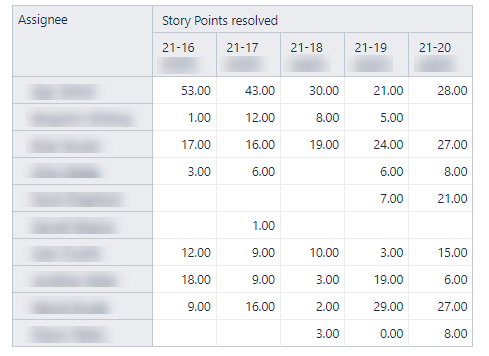# Average story points resolved by assignee over the last x sprints

How would I get the average story points resolved by assigned over the last 5 sprints? I am new to eazyBI and measures. I have built the table below, I just need an average column added to it.jloveday

Here is what you can try using eazyBI features (this method will require first creating a calculated member in “Sprint” dimension that aggregates last 5 closed sprints, then using similar filter inside the calculated measure.
Check the video:

Martins / eazyBI

p.s. you can import this definition to create this report from my video:

``````{
"cube_name": "Issues",
"cube_reports": [ {
"name": "last 5 closed sprints with average",
"result_view": "table",
"definition": {"columns":{"dimensions":[{"name":"Measures","selected_set":["[Measures].[Story Points resolved]","[Measures].[Average story points resolved]"],"members":[{"depth":0,"name":"Story Points resolved","full_name":"[Measures].[Story Points resolved]","format_string":"#,##0","annotations":{"group":"Agile","predefined":"true"},"drilled_into_dimension":"Sprint"}]},{"name":"Sprint","selected_set":["[Sprint].[Last 5 closed sprints - sprint level]"],"members":[{"depth":0,"name":"Last 5 closed sprints - sprint level","full_name":"[Sprint].[Last 5 closed sprints - sprint level]","calculated":true,"drillable":true,"expanded":true,"drilled_into":false,"removed":true}],"bookmarked_members":[]}]},"rows":{"dimensions":[{"name":"Assignee","selected_set":["[Assignee].[User].Members"],"members":[],"bookmarked_members":[]}],"nonempty_crossjoin":true},"pages":{"dimensions":[]},"options":{},"view":{"current":"table","maximized":false,"table":{}},"calculated_members":[]}
} ],
"calculated_members": [{"name":"Last 5 closed sprints - sprint level","dimension":"Sprint","formula":"Aggregate(\n    Tail(\n      Filter(\n      [Sprint].[Sprint].Members,\n      [Sprint].CurrentMember.GetBoolean(\"Closed\")),\n      5)\n)","format_string":""},{"name":"Average story points resolved","dimension":"Measures","formula":"CASE WHEN [Measures].[Story points resolved] = 0 THEN 0\nWHEN NOT IsEmpty([Measures].[Story points resolved]) THEN\nAvg(\n    Tail(\n      Filter(\n      [Sprint].[Sprint].Members,\n      [Sprint].CurrentMember.GetBoolean(\"Closed\")),\n      5),\n      [Measures].[Story points resolved]     \n)\nEND","format_string":""}]
}

``````

how would i make a calculated field that shows this but instead of the last 5 sprints, I would like to see all closed sprints since the beginning of this year

@jeffb

Try this formula when creating calculated member in “Sprint” dimension:

``````Aggregate(
Filter(
[Sprint].[Sprint].Members,
[Sprint].CurrentMember.GetBoolean("Closed")
AND
DateBetween([Sprint].CurrentMember.getdate('Complete date'),
'first day of current year',
)
)
)
``````

It would filter closed sprints completed in the current year.

Martins / eazyBI support

I imported this definition and it worked great, i have one follow up question. I would like to see the average SP completed by assignee group, how would you suggest I do this

@jeffb
Can you create a separate report without the “Assignee” dimension - to see the results by groups?
Otherwise, it would be quite strange to calculate “Avearage by assignee group” for the last 2 columns which are already average calculations by sprints
The average of averages doesn’t seem correct.

See the image below:
I created one new calculated measure that calculates the average for the group from all assignees in the sprint that have SP resolved:

``````[Measures].[Story Points resolved]
/
Count(
Filter(
[Assignee].[User].Members,
[Measures].[Story Points resolved]>0
)
)
``````

Martins / eazyBI

I am gettin syntax error while I copy past your definition as is.

@Parth_Panchal
What syntax error do you have?
Please check if you copied all lines of this formula.

Martins / eazyBI